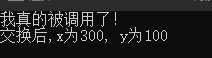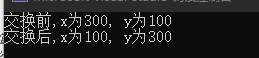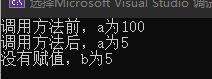C# 编程学习系列（十）方法

2、方法的组成

<访问修饰符> [static] <返回类型> <方法名>(参数类型1 参数名1,参数类型2 参数名2...)
{
方法体
}

• 访问修饰符：决定了该方法能否被其他类可见。例如：public ，是所有人可见；而 private，只能自己可见。
• static：表示静态，在某个类中是唯一的。若方法用static修饰，不需要实例化类对象，可以直接类名点方法名调用；如果没有static修饰，则需要实例化类对象来使用。
• 返回类型：决定了方法最后返回值的数据类型。这里除了 void 不需要 return，其他所有类型都需要 return 来返回值。
• 方法名：即方法的名字，当将方法写好，要调用方法的时候，可以直接通过方法名来调用。
• 参数：参数可以有多个，也可以没用。每个参数都要有对应的数据类型和参数名，参数名是在方法中调用。
• 方法体：即要实现某个任务写的代码语句。

3、方法调用实例

（1）不使用static修饰：

class c1{
static void Main(string[] args){
int n1 = 20;
int n2 = 30;
c1 c1 = new c1();
Console.WriteLine(\$"{n1}+{n2}={count}"); // 20+30=50
}

public int addNum(int num1, int num2){
int num = num1 + num2;
return num
}
}

（2）使用static修饰：

class c1{
static void Main(string[] args){
int x = 300;
int y = 100;

c2.swap(x, y);
Console.WriteLine(\$"交换后,x为{x}, y为{y}");
}
}

class c2{
public static void swap(int n1, int n2){
Console.WriteLine("我真的被调用了！");
int temp = n1;
n1 = n2;
n2 = temp;
}
}4、参数传递

（1）按引用传递参数

class c1{
static void Main(string[] args){
int x = 300;
int y = 100;

Console.WriteLine(\$"交换前,x为{x}, y为{y}");

c2.swap(ref x, ref y);
Console.WriteLine(\$"交换后,x为{x}, y为{y}");
}
}

class c2{
public static void swap(ref int n1, ref int n2){
int temp = n1;
n1 = n2;
n2 = temp;
}
}（2）按输出传递参数

return 语句可用于只从函数中返回一个值。但是，可以使用输出函数来从函数中反回两个值。输出参数会把方法输出的数据赋给自己，其他方面与引用参数相似。

class c1{
static void Main(string[] args){
c1 c1 = new c1();
int a = 100;
Console.WriteLine(\$"调用方法前，a为{a}");
c1.getValue(out a);
Console.WriteLine(\$"调用方法后，a为{a}");
c1.getValue(out int b);
Console.WriteLine(\$"没有赋值，b为{b}");
}

public void getValue(out int x){
int temp = 5;
x = temp;
}
}5、总结

（1）方法是为了减少重复代码，便于后期的阅读和维护。

（2）方法的组成：访问修饰符、返回类型、方法名以及参数类型和参数名。

（3）静态修饰符 static，可有可无。有则可以直接通过类名点方法名来调用，没有则需要将类创建一个实例化。

（4）参数传递方式：按值传递参数、按引用传递参数和按输出传递参数。

0 人点赞